In the previous MySQL tutorial on Deleting Table, you learnt how an unwanted table can be deleted from database. In this tutorial, you will learn how to select a particular data from the table using WHERE, LIKE and ORDER BY keywords.

Suppose you want a particular result from the table, you can specify that in your query using the word “where”. Let’s see this with an example of “employee” table that contains the following data as described in the [MySQL Tutorial 8] Updating Table: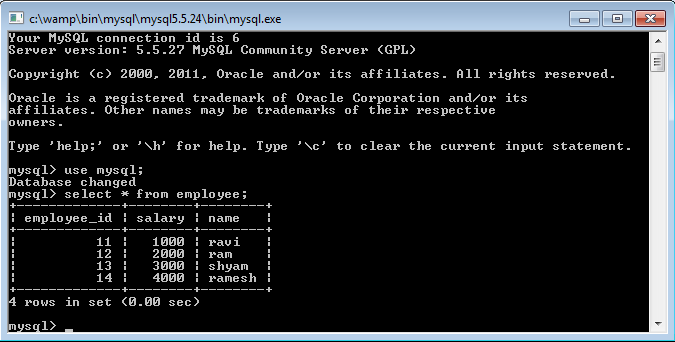The basic syntax for all queries with the use of where is:

`Select * from table_name where your_condition;`

Suppose you want to see the result only for the name “ravi” from the table “employee”, then it can be done as follows:

`Select * from employee where name=”ravi”;`

The output is as follows: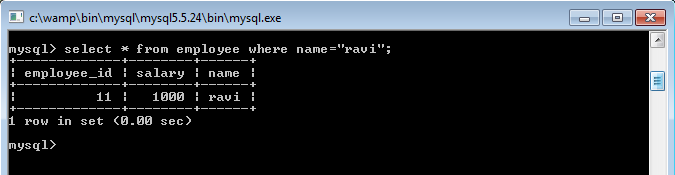Similarly, you can try for the column name as “salary” and “employee_id” also.

Let’s try some more examples but this time we’ll use delete command. The syntax for using where with delete is:

`Delete from table_name where your_condition;`

Example,

`Delete from employee where employee_id=”11”;`

The output is: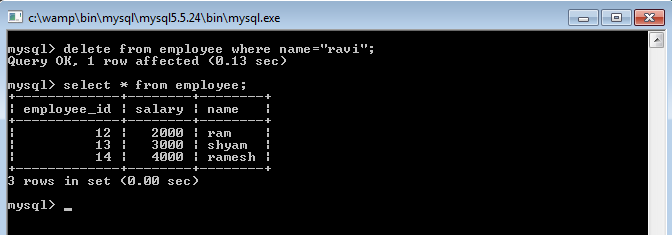Using PHP,

```<?php
mysql_select_db('mysql');
echo "connected to mysql"."<br>";
if(\$q=mysql_query('delete from employee where employee_id="11"'))
{
echo "deleted";
}
else
{
echo "not deleted";
}
?>```

The output is:Use of “where” with “update” have been described in [MySQL Tutorial 8] Updating Table.

Now we’ll see how “like” is used for querying. The syntax is:

`Select * from table_name where your_data like your_condition;`

Example,

`Select * from employee where name like “%m”;`

Here, “like” keyword searches the data in the table for the name that ends with the letter “m” and displays the output as follows: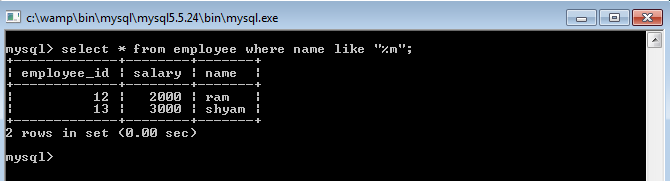Using PHP:

```<?php
mysql_select_db('mysql');
echo "connected to mysql"."<br>";
\$r=mysql_query('select * from employee where name like "%m"');
while(\$ro=mysql_fetch_array(\$r))
{
echo "employee_id:".\$ro['employee_id']."<br>";
echo "salary:".\$ro['salary']."<br>";
echo "name:".\$ro['name']."<br>";
}
?>```

The output is: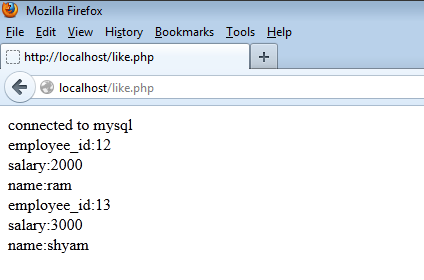What if you want the output by sorting the data, for example, by descending order, ascending order or sorted according to a particular column? In that case, ORDER BY clause is used.

Let’s take examples of how ORDER BY can be used to sort the output. We’ll use “employee” table as shown on the top of this tutorial. We see the data is in ascending order in all the columns.

To change it to descending order, the syntax is:

`Select * from table_name order by column_name desc;`

Example,

`Select * from employee order by salary desc;`

The output is: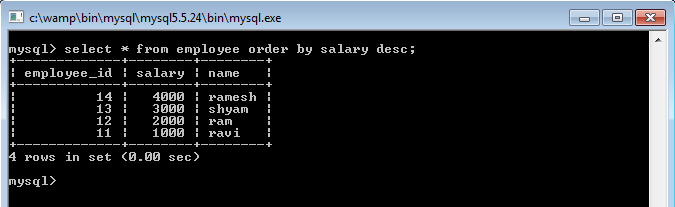`Using PHP:`
```<?php
mysql_select_db('mysql');
echo "connected to mysql<br>";
\$r=mysql_query('select * from employee order by salary desc');
while(\$ro=mysql_fetch_array(\$r))
{
echo "employee_id:".\$ro['employee_id']."<br>";
echo "salary:".\$ro['salary']."<br>";
echo "name:".\$ro['name']."<br><br>";
}
?>```

The output is: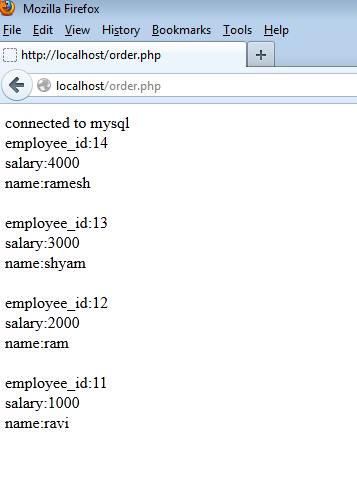Similarly, to display the output by ascending order, the syntax is:

`Select * from table_name order by column_name asc;`

Example,

`Select * from employee order by salary asc;`

We may come across with some values assigned as “null”. NULL values are not equal to zero, they simply represent the column has no value assigned. For example, in the MySQL Tutorial on Altering Table, where we added another column, the default values assigned inside the column was “null”. To draw the output having “null” as the value, the syntax is:

`Select * from table where column_name is null;`

Example,

Suppose we have a data in the “employee” table with the value of salary as null as shown below: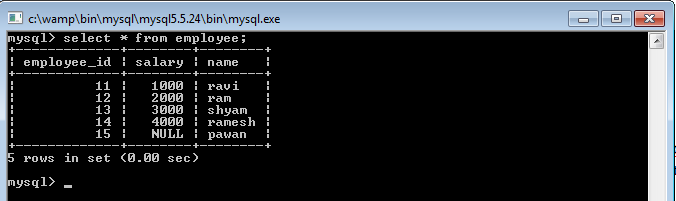Select that data as follows:

`Select * from employee where salary is null;`

The output is: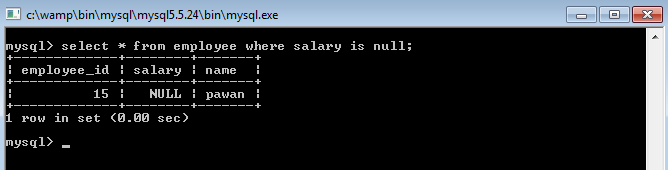Using PHP:

```<?php
mysql_select_db('mysql');
echo "connected to mysql<br>";
\$r=mysql_query('select * from employee where salary is null ');
while(\$ro=mysql_fetch_array(\$r))
{
echo "employee_id:".\$ro['employee_id']."<br>";
echo "salary:".\$ro['salary']."<br>";
echo "name:".\$ro['name']."<br><br>";
}
?>```

The output is:Now that you know how to sort result using “where”, “like”, “order by” and “null”, continue with the next tutorial on how to join the tables. Also, if you have queries, please leave a comment.

Share.